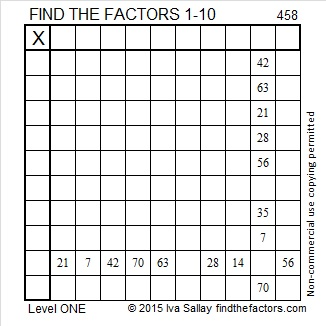# 458 and Level 1

458 = (13^2) + (17^2). It is the hypotenuse of this Pythagorean triple: 120-442-458.Print the puzzles or type the solution on this excel file:  10 Factors 2015-04-13

—————————————————————————————————

• 458 is a composite number.
• Prime factorization: 458 = 2 x 229
• The exponents in the prime factorization are 1 and 1. Adding one to each and multiplying we get (1 + 1)(1 + 1) = 2 x 2 = 4. Therefore 458 has exactly 4 factors.
• Factors of 458: 1, 2, 229, 458
• Factor pairs: 458 = 1 x 458 or 2 x 229
• 458 has no square factors that allow its square root to be simplified. √458 ≈ 21.4009—————————————————————————————————